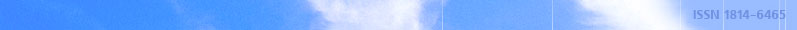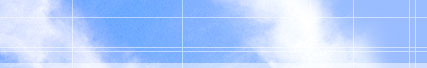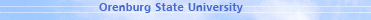december 07, 2021 RU/EN
 Headings of Vestnik Pedagogics Psychology Other

 Search Author Article

# September 2015, № 9 (184)

Gerasimenko S.A., Rustanov A.R., Shchipkova N.N. ANALOGS IDENTITIES GRAY FOR TENSOR CONHARMONIC CURVATURE AC-MANIFOLDS OF CLASS C11The main objective is to study the geometry of the curvature tensor conharmonic AC-class varieties C11. For this purpose, the following two problems are solved: 1) get contact analogues identities Gray conharmonic curvature tensor introduced in consideration of Ishi; 2) on the basis of these identities to isolate and study the subclasses of AC-class varieties C11. The paper identified three classes of almost contact metric manifolds class C11, named as GK1-, GK2- and GK3-manifolds. In Theorem 1 we obtain conditions on the curvature tensor components conharmonic on the space of the associated G-structure in which almost contact metric structure belongs to a class C11 selected classes. Theorem 2 is proved that the variety of AC-class C11 is GK3-manifold and the GK2-manifold. In Theorem 3 proved that AC-manifold of class C11, which is GK1-manifold is a manifold with Einstein's cosmological constant. In particular, in the case of completeness and continuity is compact and has a finite fundamental group. Finally, in Theorem 4 is proved that the variety of AC-class C11 dimension greater than 5 is GK1-manifold if and only if it is Ricci flat manifold.Key words: identities Gray, the Riemann-Christoffel tensor, the Ricci tensor, the tensor conharmonic curvature.

References:

1. Rustanov A.R., Shchipkova N.N. Differential geometry of almost contact metric manifolds of class C11. // Vestnik OSU, № 9 (115), September 2010, p. 65-68

2. Rustanov A.R., Shchipkova N.N. Curvature identities of varieties of class C11. // Vestnik OSU, number 6 (125), June 2011, p. 169-171.

3. Rustanov A.R., Shchipkova N.N. The geometry of the curvature tensor Concircular AC-manifolds of class C11. // Vestnik OSU, number 9 (170), September 2014, p. 114-120.

4. Rustanov A.R., Shchipkova N.N. The geometry of Weyl conformal curvature tensor AC-manifolds of class C11. // Actual problems of mathematics and related matters. Proceedings of the international scientific conference "Muhtarovskie read." April 22-23. Makhachkala, 2014, p. 89-96.

5. Chinea D., Gonzalez C. Classification of almost contact metric structures. // Annali di Matematica pura ed applicata (IV), v. CLVI, 1990, p. 15–36.

6. Gray A. Curvature identities for Hermitian and almost Hermitian manifolds. // Tohoku Math. J., 1976, v.28, p. 601-612.

7. Ishii Y. On conharmonic transformations. // Tensor, 7(2), 1957, 73-80.

8. U.C. De, R.N. Singh, Shravan K. Pandey. On the Conharmonic Curvature Tensor of Generalized Sasakian-Space-Forms. // International Scholarly Research Network ISRN Geometry. Volume 2012, Article ID 876276, 14 pages doi:10.5402/2012/876276.

9. V.F. Kirichenko, A.A. Shihab. On the geometry of conharmonic curvature tensor for nearly Kдhler manifolds. // Journal of Mathematical Sciences, September 2011, Volume 177, Issue 5, pp 675-683.

10. Mohit Kumar Dwivedi, Jeong-Sik Kim. On Conharmonic Curvature Tensor in K-contact and Sasakian Manifolds. // Bull. Malays. Math. Sci. Soc. (2) 34(1) (2011), 171–180. http://math.usm.my/bulletin

11. V. F. Kirichenko, A. R. Rustanov, A. A. Shikhab, Geometry of the Conharmonic Curvature Tensor of Almost Hermitian Manifolds, Mat. Zametki, 2011, Volume 90, Issue 1, 87–103. http://www.mathnet.ru/eng/agreement

12. Ali Akbar, Avijit Sarkar, On the Conharmonic and Concircular curvature tensors of almost C(λ) manifolds, International Journal of Advanced Mathematical Sciences (IJMSEA), 1:3 (2013), 134-138.

13. Ali A. Shihab. On the geometry of conharmonic curvtuar tensor of nearly kahler manifold. // Journal of Basrah Researches ((Sciences)) Volume 37. Number 4 C ((2011)), 39 — 48.

14. Ghosh Sujit; De U.C.; Taleshian A. Conharmonic Curvature Tensor on N(K)-Contact Metric Manifolds // ISRN Geometry;2011, Special section p1.

15. Kharitonova S.V. Tensor of normal curvature kongarmonicheskoy locally conformally nearly cosymplectic varieties // Vestnik OSU № 12 (161) / 2013, 182 — 186.Editor-in-chief Sergey Aleksandrovich MIROSHNIKOV